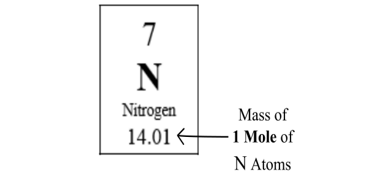# All Topics     |   Breslyn.org   |     Stoichiometry Topics

### How to Find Molar Mass

In this video you'll learn calculate the molar mass (sometimes called molecular weight and gram formula mass). The Periodic Table provides a list of molar masses for all elements.

### How to Find Molar Mass

• You must have a Periodic Table to find molar mass (unless you memorize the masses of all the elements).

• The units are grams/mole or g/mol. This is important to include in your final answer.

• The reason Molar Mass is so important is that it allows us to convert from grams to moles.

More Help and Practice:

### Finding Molar MassThe Periodic Table provides the mass (grams) of one mole of each element. For Nitrogen (N) one mole of N atoms has a mass of 14.01 grams.

Go To Top
Subscribe